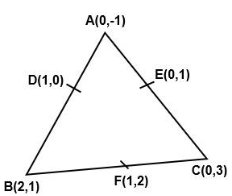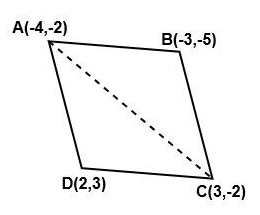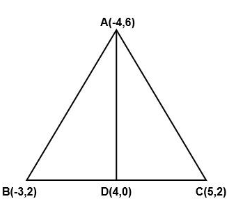Courses
Courses for Kids
Free study material
Offline Centres
More

# NCERT Solutions for Class 10 Maths Chapter 7 - ExerciseLast updated date: 03rd Dec 2023
Total views: 532.8k
Views today: 9.32k

## NCERT Solutions for Class 10 Maths Chapter 7 Coordinate Geometry (Ex 7.3) Exercise 7.3

Watch videos on
NCERT Solutions for Class 10 Maths Chapter 7 - ExerciseCOORDINATE GEOMETRY in One Shot (Complete Chapter) | CBSE 10 Maths Chapter 6 - Term 1 Exam | Vedantu
Vedantu 9&10
SubscribeShare
6.1K likes
116K Views
2 years agoCOORDINATE GEOMETRY L-1 [Distance Formula] CBSE Class 10 Maths Chapter 7 (Term 1 Exam) NCERT Vedantu
Vedantu 9&10
8.1K likes
112.6K Views
2 years agoCoordinate Geometry in One Shot | CBSE Class 10 Maths Chapter 7 NCERT Solutions | Vedantu
Vedantu 9&10
11.2K likes
313.6K Views
3 years agoCoordinate Geometry L1 | Distance Formula | CBSE Class 10 Maths | NCERT Solutions | Umang Vedantu
Vedantu 9&10
9.1K likes
216.8K Views
3 years ago

## Access NCERT solutions for Maths Chapter 7 – Coordinate Geometry

EXERCISE NO: 7.3

1. Find the area of the triangle whose vertices are: $\text{(2, 3), (- 1, 0), (2, - 4) }$

Ans: Assume A$({{x}_{1}},\text{ }{{y}_{1}})=\left( 2,\text{ }3 \right)$ , B$\left( {{x}_{2}},\text{ }{{y}_{2}} \right)=\left( -1\text{ },\text{ }0 \right)$ , C$\left( {{x}_{3}},\text{ }{{y}_{3}} \right)=\left( 2,-4 \right)$

Area of a triangle =$\frac{1}{2}\left\{ {{x}_{1}}({{y}_{2}}-{{y}_{3}})+{{x}_{2}}({{y}_{3}}-{{y}_{1}})+{{x}_{3}}({{y}_{1}}-{{y}_{2}}) \right\}$

By putting the above values of A,B,C and D

We get,

=$\frac{1}{2}\left\{ 2(0-(-4))+(-1)(-4-3)+2(3-0) \right\}$

=$\frac{1}{2}\left\{ 8+7+6 \right\}$

=$\frac{21}{2}$ square units

2. Find the area of the triangle whose vertices are: $\text{(-5, -1), (3, -5), (5, 2) }$

Ans: Assume A$({{x}_{1}},\text{ }{{y}_{1}})=\left( -5,-1 \right)$,B$\left( {{x}_{2}},\text{ }{{y}_{2}} \right)=\left( \text{3,-5} \right)$ , C$\left( {{x}_{3}},\text{ }{{y}_{3}} \right)=\left( 5,2 \right)$

Area of a triangle =$\frac{1}{2}\left\{ {{x}_{1}}({{y}_{2}}-{{y}_{3}})+{{x}_{2}}({{y}_{3}}-{{y}_{1}})+{{x}_{3}}({{y}_{1}}-{{y}_{2}}) \right\}$

By putting the above values of A,B,C and D

We get,

=$\frac{1}{2}\left\{ (-5)((-5)-(2))+3(2-(-1))+5(-1-(-5) \right\}$

=$\frac{1}{2}\left\{ 35+9+20 \right\}$

=$32$square units

3 (i) In each of the following find the value of ‘k’, for which the points are collinear:$\text{(7, - 2), (5, 1), (3, - k})$

Ans: Assume A$({{x}_{1}},\text{ }{{y}_{1}})=\left( 7,-2 \right)$,B$\left( {{x}_{2}},\text{ }{{y}_{2}} \right)=\left( 5,1 \right)$ , C$\left( {{x}_{3}},\text{ }{{y}_{3}} \right)=\left( 3,-k \right)$

Area of a triangle for collinear point$\frac{1}{2}\left\{ {{x}_{1}}({{y}_{2}}-{{y}_{3}})+{{x}_{2}}({{y}_{3}}-{{y}_{1}})+{{x}_{3}}({{y}_{1}}-{{y}_{2}}) \right\}=0$

By putting the above values of A,B,C and D

We get,

=$\frac{1}{2}\left\{ 7(1+k)+5(2-k)+3((-2)-1) \right\}=0$

=$7+7k-5k+10-9=0$

=$2k+8=0$

=$k=-4$

As a result, the supplied points for $k=-4$ are collinear.

3 (ii) In each of the following find the value of ‘k’, for which the points are collinear:$\text{(8, 1), (k, - 4), (2, - 5)}$

Ans: Assume A$({{x}_{1}},\text{ }{{y}_{1}})=\left( 8,1 \right)$,B$\left( {{x}_{2}},\text{ }{{y}_{2}} \right)=\left( k,-4 \right)$ , C$\left( {{x}_{3}},\text{ }{{y}_{3}} \right)=\left( 2,-5 \right)$

Area of a triangle for collinear point$\frac{1}{2}\left\{ {{x}_{1}}({{y}_{2}}-{{y}_{1}})+{{x}_{2}}({{y}_{3}}-{{y}_{1}})+{{x}_{3}}({{y}_{1}}-{{y}_{2}}) \right\}=0$

By putting the above values of A,B,C and D

We get,

=$\frac{1}{2}\left\{ 8(-4-(-5)+k((-5)-(1))+2(1-(-4) \right\}=0$

=$8-6k+10=0$

=$6k=18$

=$k=3$

As a result, the supplied points for $k=3$ are collinear.

4. Find the area of the triangle formed by joining the mid-points of the sides of the triangle whose vertices are $(0,-1),(2,1)$ and $(0,3)$.Find the ratio of this area to the area of the given triangle.

Ans:Assume A$({{x}_{1}},{{y}_{1}})=(0,-1)$,B$\left( {{x}_{2}},\text{ }{{y}_{2}} \right)=\left( 2,1 \right)$ , C$\left( {{x}_{3}},\text{ }{{y}_{3}} \right)=\left( 0,3 \right)$

Area of a triangle =$\frac{1}{2}\left\{ {{x}_{1}}({{y}_{2}}-{{y}_{1}})+{{x}_{2}}({{y}_{3}}-{{y}_{1}})+{{x}_{3}}({{y}_{1}}-{{y}_{2}}) \right\}$

Let D, E, and F be the midpoints of the triangle's sides. D, E, and F coordinates are determined by :

$D=\left( \frac{0+2}{2},\frac{-1+1}{2} \right)=(1,0)$

$E=\left( \frac{0+0}{2},\frac{3-1}{2} \right)=(0,1)$

$F=\left( \frac{2+0}{2},\frac{1+3}{2} \right)=(1,2)$

By putting the above values we get area of triangle DEF

=$\frac{1}{2}\left\{ (2-1)+1(1-0)+0(0-2) \right\}$

=$\frac{1}{2}(1+1)=1$Square units

Similarly,

Area of triangle ABC=$\frac{1}{2}\left\{ 0(1-3)+2(3-(-1)+0(-1-1) \right\}$

=$\frac{1}{2}(8)=4$Square units.

As a result, Ratio of this area ∆DEF to the area of the triangle ∆ABC= $1:4$

5. Find the area of the quadrilateral whose vertices, taken in order, are $(-4,-2),(-3,-5),(3,-2)$ and $(2,3)$.

Ans:Let the quadrilateral's vertices be A $(4,2)$ , B$(3,5)$ , C $(3,-2)$, and D $(3,2)$.

Form two triangles, ABC and ACD, by joining AC.

Area of a triangle =$\frac{1}{2}\left\{ {{x}_{1}}({{y}_{2}}-{{y}_{1}})+{{x}_{2}}({{y}_{3}}-{{y}_{1}})+{{x}_{3}}({{y}_{1}}-{{y}_{2}}) \right\}$

By putting the values of A,B,C in the above formula we get:

=$\frac{1}{2}\left\{ (-4)((-5)-(-2)+(-3)((-2)-(-2))+3((-2)-(-2) \right\}$

=$\frac{1}{2}(12+0+9)=\frac{21}{2}$Square units

By putting the values of A,C,D in the above formula we get:

=$\frac{1}{2}\left\{ (-4)((-2)-(-3)+3(3-(-2))+2((-2)-(-2) \right\}$

=$\frac{1}{2}(20+15+0)=\frac{35}{2}$Square units

So, Area of  triangle ABCD= Area of triangle ABC + Area of triangle ACD

=$\left( \frac{21}{2}+\frac{35}{2} \right)=28$ Square units

6. You have studied in Class IX that a median of a triangle divides it into two triangles of equal areas. Verify this result for ∆ABC whose vertices are A $(4,-6)$ , B $(3,-2)$  and C $(5,2)$

Ans:The triangle's vertices should be A (4, 6), B (3, 2), and C (5, 2).

Assume D is the midpoint of ABC's side BC.

As a result, in ABC, AD is the median.

D point coordinates are:$\left( \frac{3+5}{2}+\frac{-2+2}{2} \right)=(4,0)$

Area of a triangle =$\frac{1}{2}\left\{ {{x}_{1}}({{y}_{2}}-{{y}_{1}})+{{x}_{2}}({{y}_{3}}-{{y}_{1}})+{{x}_{3}}({{y}_{1}}-{{y}_{2}}) \right\}$

By putting the values of A,B,D in the above formula we get:

Area of triangle ABD=$\frac{1}{2}\left\{ (-4)((-2)-(0)+3(0-(-6))+4((-6)-(-2) \right\}$

=$\frac{1}{2}(-8+18-16)=-3$Square units

By putting the values of A,B,D in the above formula we get:

Area of triangle ADC=$\frac{1}{2}\left\{ (4)(0-(-2)+4(2-(-6))+5((-6)-(0) \right\}$

=$\frac{1}{2}(-8+32-30)=-3$Square units

As we know area cannot be in negative. So, Area of triangle ADC is 3 Square units.

As a result, median AD has clearly separated triangle ABC into two equal-sized triangles.

## NCERT Solutions for Class 10 Maths Chapter 7 Coordinate Geometry Exercise 7.3

Opting for the NCERT solutions for Ex 7.3 Class 10 Maths is considered as the best option for the CBSE students when it comes to exam preparation. This chapter consists of many exercises. Out of which we have provided the Exercise 7.3 Class 10 Maths NCERT solutions on this page in PDF format. You can download this solution as per your convenience or you can study it directly from our website/ app online.

Vedantu in-house subject matter experts have solved the problems/ questions from the exercise with the utmost care and by following all the guidelines by CBSE. Class 10 students who are thorough with all the concepts from the Maths textbook and quite well-versed with all the problems from the exercises given in it, then any student can easily score the highest possible marks in the final exam. With the help of this Class 10 Maths Chapter 7 Exercise 7.3 solutions, students can easily understand the pattern of questions that can be asked in the exam from this chapter and also learn the marks weightage of the chapter. So that they can prepare themselves accordingly for the final exam.

Besides these NCERT solutions for Class 10 Maths Chapter 7 Exercise 7.3, there are plenty of exercises in this chapter which contain innumerable questions as well. All these questions are solved/answered by our in-house subject experts as mentioned earlier. Hence all of these are bound to be of superior quality and anyone can refer to these during the time of exam preparation. In order to score the best possible marks in the class, it is really important to understand all the concepts of the textbooks and solve the problems from the exercises given next to it.

Do not delay any more. Download the NCERT solutions for Class 10 Maths Chapter 7 Exercise 7.3 from Vedantu website now for better exam preparation. If you have the Vedantu app in your phone, you can download the same through the app as well. The best part of these solutions is these can be accessed both online and offline as well.

## FAQs on NCERT Solutions for Class 10 Maths Chapter 7 - Exercise

1. What are the topics and subtopics covered in Class 10 Maths Chapter 7?

Ans: Class 10 Maths Chapter 7 deals with Coordinate Geometry and it contains three subtopics which are given below.

• Introduction to Coordinate Geometry.

• Distance Formula.

• Section Formula.

• Area of a Triangle.

2. How many chapters are contained in the Class 10th Maths syllabus?

Ans: There are a total of 15 chapters. Take a look below.

• Chapter 1: Real Numbers.

• Chapter 2: Polynomials.

• Chapter 3: Pair of linear Equations in Two Variables.

• Chapter 5: Arithmetic Progression.

• Chapter 6: Triangles.

• Chapter 7: Coordinate Geometry.

• Chapter 8: Introduction to Trigonometry.

• Chapter 9: Some Applications of Trigonometry.

• Chapter 10: Circles.

• Chapter 11: Constructions.

• Chapter 12: Areas Related to Circles.

• Chapter 13: Surface Areas and Volumes.

• Chapter 14: Statistics.

• Chapter 15: Probability.

3. How many questions are there Class 10 Maths Chapter 7 Exercise 7.3?

Ans: Five questions are there in total in the Exercise 7.3 of Class 10 Maths Chapter 7. Solutions to all these questions are also provided in the NCERT Solutions of Class 10 Maths Chapter 7 Exercise 7.3. These solutions are created by top-notch experts from the relevant field in an accurate manner.

Ans: Free PDF download of Class 10 Maths Chapter 7 Exercise 7.3 NCERT Solutions along with all chapters’ exercises are available on Vedantu which are prepared by the experts. These are created as per NCERT (CBSE) curriculum and guidelines.

Class 10 Maths Chapter 7 Coordinate Geometry Exercise 7.3 NCERT textbook questions with Solutions help you to revise the entire syllabus in a very less time and score the highest possible marks. Register on Vedantu and get solutions for all the exercises of  Class 10 Maths Chapter 7 NCERT textbook. You can also download other relevant study materials to score more marks in the Class 10th board examination.

5. How to find the area of a triangle whose vertices are given according to Chapter 7 of Class 10 Maths?

Ans: To find the area of a triangle whose vertices are given, you use the formula: ½ [x1 ( y2 - y3) + x2 (y3 - y1) + x3 (y1 - y2)], where the coordinates of the three vertices are (x1, y1), (x2, y2), and (x3, y3). By applying the values of the coordinates in the formula given above, you can easily calculate the area of a given triangle. Get your hands on Vedantu’s NCERT Solutions of Exercise 7.3 of Chapter 7 of Class 10 Maths for complete solutions.

6. Which is the best example for Exercise 7.3 of Chapter 7 of Class 10 Maths?

Ans: Examples 11 to 15 that are given in the NCERT Chapter 7 of Class 10 Maths are the best examples that will help you in solving all the questions that have been asked in Exercise 7.3.  If you need further guidance on solving the questions from this exercise, you must choose the NCERT Solutions Exercise 7.3 of Chapter 7 of Class 10 Maths available on Vedantu. All explanations are provided by experts and each answer is given step-wise and can be downloaded free of cost.

7. Is Exercise 7.3 of Chapter 7 of Class 10 Maths easy?

Ans: Yes, Exercise 7.3 of Chapter 7 of Class 10 Maths is easy for those who have completed all the previous exercises that are there in the chapter and have also practised all the examples for a very strong base and understanding of concepts. You can take the help of the best NCERT Solutions that you will find in the market today. Vedantu’s NCERT Solutions for Exercise 7.3 of Chapter 7 of Class 10 Maths is the best. You can visit Vedantu to get access to expert answers.

8. What are the basic concepts taught in Exercise 7.3 of Chapter 7 of Class 10 Maths?

Ans: Exercise 7.3 of Chapter 7 " Coordinate Geometry" of Class 10 Maths is a significant exercise based on the following concept:

Area of a Triangle = 1/2 × base × altitude

NCERT textbook has provided 5 examples to explain this concept more clearly. Exercise 7.3 tests the students’ understanding of this concept and the examples provided. Students must practice the examples before attempting the main exercise. When you attempt Exercise 7.3, you can visit Vedantu's NCERT Solutions for Class 10 Maths Exercise 7.3 for reference.

9. What other study aids can Vedantu provide apart from NCERT Solutions, to practice Exercise 7.3 of Chapter 7 of Class 10 Maths efficiently?

Ans: Added to the NCERT Solutions, our experts also work tirelessly to provide several additional study materials to help you prepare meticulously for your CBSE Board examinations. These include:

1. Revision notes

2. Important questions

3. Solved sample papers and previous years questions papers

4. Exemplar solutions

5. Reference book solutions for books like RS Agarwal, RD Sharma, Lakhmir Singh, etc.

6. Conceptual videos

7. Live masterclasses

8. Personal one-on-one classes

If you make efficient use of these tools, you are sure to ace your exams.

NOTE: Plagiarism shown is due to the formula of the area of a triangle.